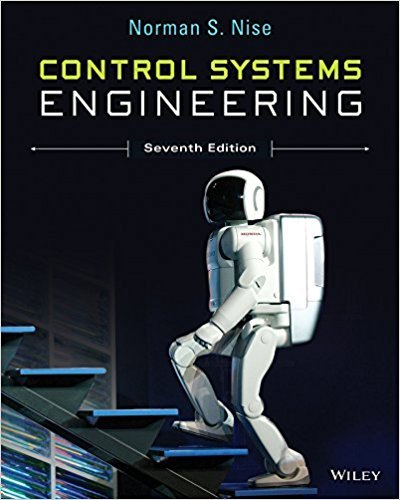×
Get Full Access to Control Systems Engineering - 7 Edition - Chapter 3 - Problem 24
Get Full Access to Control Systems Engineering - 7 Edition - Chapter 3 - Problem 24

×

# In this chapter, we described the state-spaceISBN: 9781118170519 162

## Solution for problem 24 Chapter 3

Control Systems Engineering | 7th Edition

• Textbook Solutions
• 2901 Step-by-step solutions solved by professors and subject experts
• Get 24/7 help from StudySoup virtual teaching assistantsControl Systems Engineering | 7th Edition

4 5 1 381 Reviews
20
2
Problem 24

In this chapter, we described the state-space representation of single-input, single-output systems. In general, systems can have multiple inputs and multiple outputs. An autopilot is to be designed for a submarine as shown in Figure P3.15 to maintain a constant depth under severe wave disturbances. We will see that this system has two inputs and two outputs and thus the scaler u becomes a vector, u, and the scaler y becomes a vector, y, in the state equations.It has been shown that the systems linearized dynamics under neutral buoyancy and at a given constant speed are given by (Liceaga-Castro, 2009): x_ Ax Bu y Cx where x w q z 2 6 6 6 6 4 3 7 7 7 7 5 ; y z " #; u B S " # A 0:038 0:896 0 0:0015 0:0017 0:092 0 0:0056 1 00 3:086 0 1 00 2 6 6 6 6 6 6 4 3 7 7 7 7 7 7 5 ; B 0:0075 0:023 0:0017 0:0022 0 0 0 0 2 6 6 6 6 6 6 4 3 7 7 7 7 7 7 5 ; C 0010 0001 " # and where w the heave velocity q the pitch rate z the submarine depth the pitch angle B the bow hydroplane angle S the stern hydroplane angle Since this system has two inputs and two outputs, four transfer functions are possible. a. Use MATLAB to calculate the systems matrix transfer function. b. Using the results from Part a, write the transfer function zs Bs ; zs Ss ; s Bs ; and s Ss .

Step-by-Step Solution:
Step 1 of 3

CS 6505|Section O01 Homework 0 Fall 2015 Directions: This problem set covers background material for the course: logic, set theory, induction, and asymptotic analysis. You may ▯nd it useful to consult Ken Rosen’s textbook Discrete Math and Its Applications. Your answers should be in complete sentences. You may use truth-tables where appropriate. This assignment is due on Monday, August 24, at 8:00 a.m. U.S. Eastern Time. Turn in your answers as a PDF ▯le (not a Word document) in the Assignments section of T-Square. 1. (1 point) (a) Give the negation and the contrapositive of the following statement: \If every bird ies, then there is a hungry cat." (b) A proposition is a statement that ca

Step 2 of 3

Step 3 of 3

##### ISBN: 9781118170519

This textbook survival guide was created for the textbook: Control Systems Engineering, edition: 7. The answer to “In this chapter, we described the state-space representation of single-input, single-output systems. In general, systems can have multiple inputs and multiple outputs. An autopilot is to be designed for a submarine as shown in Figure P3.15 to maintain a constant depth under severe wave disturbances. We will see that this system has two inputs and two outputs and thus the scaler u becomes a vector, u, and the scaler y becomes a vector, y, in the state equations.It has been shown that the systems linearized dynamics under neutral buoyancy and at a given constant speed are given by (Liceaga-Castro, 2009): x_ Ax Bu y Cx where x w q z 2 6 6 6 6 4 3 7 7 7 7 5 ; y z " #; u B S " # A 0:038 0:896 0 0:0015 0:0017 0:092 0 0:0056 1 00 3:086 0 1 00 2 6 6 6 6 6 6 4 3 7 7 7 7 7 7 5 ; B 0:0075 0:023 0:0017 0:0022 0 0 0 0 2 6 6 6 6 6 6 4 3 7 7 7 7 7 7 5 ; C 0010 0001 " # and where w the heave velocity q the pitch rate z the submarine depth the pitch angle B the bow hydroplane angle S the stern hydroplane angle Since this system has two inputs and two outputs, four transfer functions are possible. a. Use MATLAB to calculate the systems matrix transfer function. b. Using the results from Part a, write the transfer function zs Bs ; zs Ss ; s Bs ; and s Ss .” is broken down into a number of easy to follow steps, and 270 words. The full step-by-step solution to problem: 24 from chapter: 3 was answered by , our top Engineering and Tech solution expert on 11/23/17, 05:05AM. Since the solution to 24 from 3 chapter was answered, more than 347 students have viewed the full step-by-step answer. This full solution covers the following key subjects: Systems, outputs, inputs, angle, transfer. This expansive textbook survival guide covers 13 chapters, and 734 solutions. Control Systems Engineering was written by and is associated to the ISBN: 9781118170519.

Unlock Textbook Solution